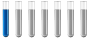## Forsterite (olivine) dissolution reaction / assumptions

Chemistry and homework help forum.

Organic Chemistry, Analytical Chemistry, Biochemistry, Physical Chemistry, Computational Chemistry, Theoretical Chemistry, High School Chemistry, Colledge Chemistry and University Chemistry Forum.

Share your chemistry ideas, discuss chemical problems, ask for help with scientific chemistry questions, inspire others by your chemistry vision!

Please feel free to start a scientific chemistry discussion here!

Discuss chemistry homework problems with experts!

Ask for help with chemical questions and help others with your chemistry knowledge!

Moderators: expert, ChenBeier, Xen

pbuis
NewbiePosts: 1
Joined: Fri Mar 05, 2021 7:39 am

### Forsterite (olivine) dissolution reaction / assumptions

I am studying dissolution of forsterite (olivine) at ambient temperature and pressure:

Mg2SiO4 + 4H2O + 4 CO2 ------ H4 SiO4 + 4 HCO3- + 2 Mg 2+ (reaction A)

I used the assumptions listed hereunder to estimate the concentration in Mg 2+ based on pH measurement (9,8) but the result is completely inconsistent with the prediction based on the kinetic model. I assume the assumptions I used may not be valid. Could someone tell me which of the reactions (1) to (9) I used might not be valid ?
a/ In the table hereunder I listed the various chemical species I take into account and the associated concentrations (“a” = concentration in CO2 aq, etc…).
CO2 aq a
HCO3- b
CO3 2- c
H+
Mg2+ d
H4SiO4 e
Si02 f
H3SiO4- g
H2SiO42- h
Tot Si e + f + g + h
OH- k

b/ I used the equilibrium relations (1) to (8) and equation (9) (based on Mg 2+ to Si ratio resulting from reaction (A)):

(1) CO2 aq with atmospheric CO2 g (allows to obtain “a” concentration in CO2 aq / K0 = 0, 0396)
(2) CO2 aq with HCO3 - / K1 = 4,2E-07 (allows to obtain “b” concentration in HCO3- knowing “a”)
(3) HCO3 – with CO3 2- / K2 = 4,3E-11 (allows to obtain “c” concentration in CO3 2- knowing “b”)
(4) SiO2(s) + 2H2O = H4SiO4 (aq) ; K1a = 10-2,7
(5) H4SiO4 (aq) + H2O = H3SiO4- (aq) + H3O+(aq) K2a = 3,162E-10
(6) H3SiO4- (aq) + H2O = H2SiO4 2- + H3O+(aq) / K3a = 2,512E-13
(7) Electrical equilibrium : b + 2c + g + 2h + k = [H+] + 2d
(8) [H+] * [OH -] = Kw = 1E-14
(9) Based on reaction (A) we know that d= 2Tot Si = 2(e+f+g+h) since (A) produces 2 Mg2+ for 1 H4 SiO4

I am not sure how I can use the fact that (A) produces 4 HCO3- for 2 Mg 2+ ???

c/ results: based on these equations / equilibrium (1) to (9) I obtain the following :
CO2 aq a 1,4E-05 based on (1) with PCO2=0,00035 atm
HCO3- b 3,6E-02 based on (2)
CO3 2- c 9,9E-03 based on (3)
H+ 1,6E-10 based on measured pH
Mg2+ d unknown
H4SiO4 e =g/2 based on (5)
Si02 f can be neglected based on (4)
H3SiO4- g unknown
H2SiO42- h can be neglected based on (6)
OH- k 6,3E-05 based on pH and (8)
Tot Si e + f + g + h
from reaction A d=2(e+f+g+h)≈2(g+g/2)=3g based on (9)

electrical equilibrium 2d=b+2c+g+k then 6g=b+2c+g+k then 5g=b+2c+k
electrical equilibrium (contd) then g= 1,1E-02 and d= 3,4E-02

I obtain a concentration “d” in Mg 2+ (3,4E-02) which is much higher (by a factor 100) than what predicts the kinetic model. Could someone tell me if the equations (1) to (9) I used are valid in this context ? Particularly the equations allowing to compute concentration in HCO3 – et CO3 2- ?
NB : I used extra pure water to conduct the experiment therefore the initial concentration in Mg 2+ in the water was extremely low.